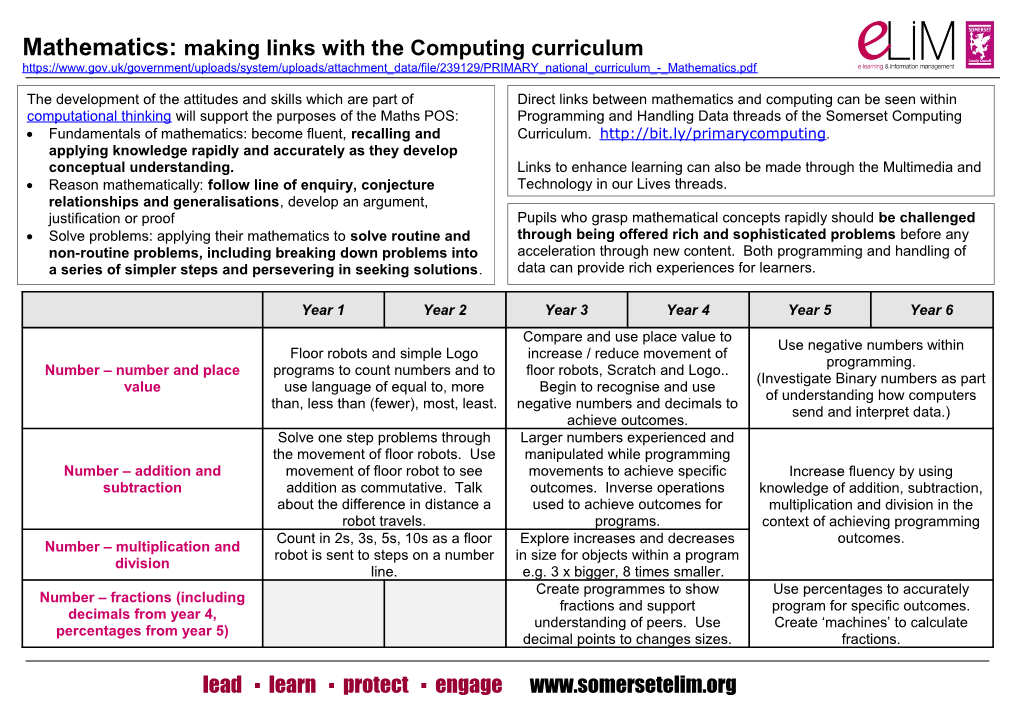# Maths and ComputingMathematics: making links with the Computing curriculumYear 1 / Year 2 / Year 3 / Year 4 / Year 5 / Year 6
Number – number and place value / Floor robots and simple Logo programs to count numbers and to use language of equal to, more than, less than (fewer), most, least. / Compare and use place value to increase / reduce movement of floor robots, Scratch and Logo.. Begin to recognise and use negative numbers and decimals to achieve outcomes. / Use negative numbers within programming.
(Investigate Binary numbers as part of understanding how computers send and interpret data.)
Number – addition and subtraction / Solve one step problems through the movement of floor robots. Use movement of floor robot to see addition as commutative. Talk about the difference in distance a robot travels. / Larger numbers experienced and manipulated while programming movements to achieve specific outcomes. Inverse operations used to achieve outcomes for programs. / Increase fluency by using knowledge of addition, subtraction, multiplication and division in the context of achieving programming outcomes.
Number – multiplication and division / Count in 2s, 3s, 5s, 10s as a floor robot is sent to steps on a number line. / Explore increases and decreases in size for objects within a program e.g. 3 x bigger, 8 times smaller.
Number – fractions (including decimals from year 4, percentages from year 5) / Create programmes to show fractions and support understanding of peers. Use decimal points to changes sizes. / Use percentages to accurately program for specific outcomes. Create ‘machines’ to calculate fractions.
Measurement / Floor robots and simple Logo programs to solve practical problems for length and height. / Investigate the perimeter of shapes through programming. Create a timer for particular tasks / games. / Create conversion ‘machines’ and scaling ‘machines’.
Geometry – properties of shape / Floor robots and simple Logo programs to create rectangles. Use simple software to print out nets and create 3D shapes. / Recognises the amount of turn required to create 2-d shapes as they are drawn with floor robots, Logo or Scratch.
Investigate right angles.
Use the vocabulary of properties of shapes when created objects and patterns. Program nets to create 3D shapes. / Program with appropriate software to create regular and irregular polygons. Use the language of angles while programming letters and shapes.
Geometry – position and direction / Floor robots and simple Logo programs to describe direction and movement, including whole, half, quarter and three-quarter turns. / Use the coordinates with Scratch software to create movement. / Explore reflection and translation with appropriate programming software.
Statistics / Construct and interpret pictograms and block diagrams. / Use appropriate software and apps to present and interpret data. Use the information to solve one and two step problems. Interpret data collected with data loggers. / Use appropriate software and data loggers to create and interpret line graphs. Complete and interpret tables to present and understand information.
Ratio and proportion (year 6) / Create Pie charts and interpret number of degrees for different data. Use Scratch and Logo to explore proportionate increases and decreases to size of images and shapes.
Algebra (year 6) / Use operator blocks in Scratch to build understanding of symbols. Create missing number ‘machines’.

For mathematical challenges with FMS Logo see Programming page of Somerset Primary Computing Curriculum.

For mathematical challenges with Scratch see Scratch Programming page (key development tools). Also Maths Cat (support sheet 4a).

lead ▪ learn ▪ protect ▪ engage